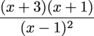Study Guide

# Multiplying and Dividing Rational Expressions

## Multiplying and Dividing Rational Expressions

We multiply and divide rational expressions just like fractions. Wait—is this thing on repeat? To multiply rational expressions, first factor all numerators and denominators and cancel any factors you can. Then multiply what you have left. To divide, simply flip the divisor (the term you're dividing by) and then multiply. In math-speak, it's called multiplying by the reciprocal of the divisor. Yikes.

### Sample Problem

Multiply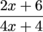by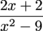.

Factor your numerators and denominators as much as you possibly can.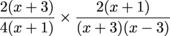Next, cancel where possible.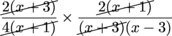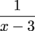Not too awful, right?

### Sample Problem

Multiply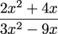by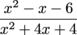.

Break everything down into factors. Don't hold back.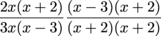Cancel like factors.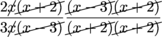Which gives us: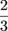### Sample Problem

Simplify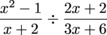.

First flip the divisor over and multiply.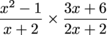Now factor the numerators and denominators.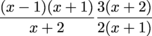Cancel like factors.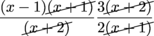And we get: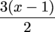### Sample Problem

Divide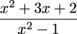by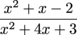.

Flip the divisor over and multiply.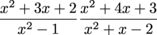Factor the numerators and denominators.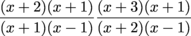Go on a huge canceling spree.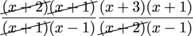Then keep all the leftover stuff to get our final answer: